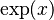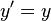# Special functions facts for kids

Kids Encyclopedia Facts

Special functions are some mathematical functions used in mathematical analysis or physics. Most of them appear in higher education. Some experts are studying numerical methods for them.

## Definition

In mathematics, most functions are defined as a solution of a differential equation. For example, the exponential function$\exp(x)$ is the solution of the ordinary differential equation$y^\prime=y$. Due to this relation, some mathematicians are studying the connection between ODEs and special functions.

## Examples

For more examples, find textbooks named "special functions".Special functions Facts for Kids. Kiddle Encyclopedia.